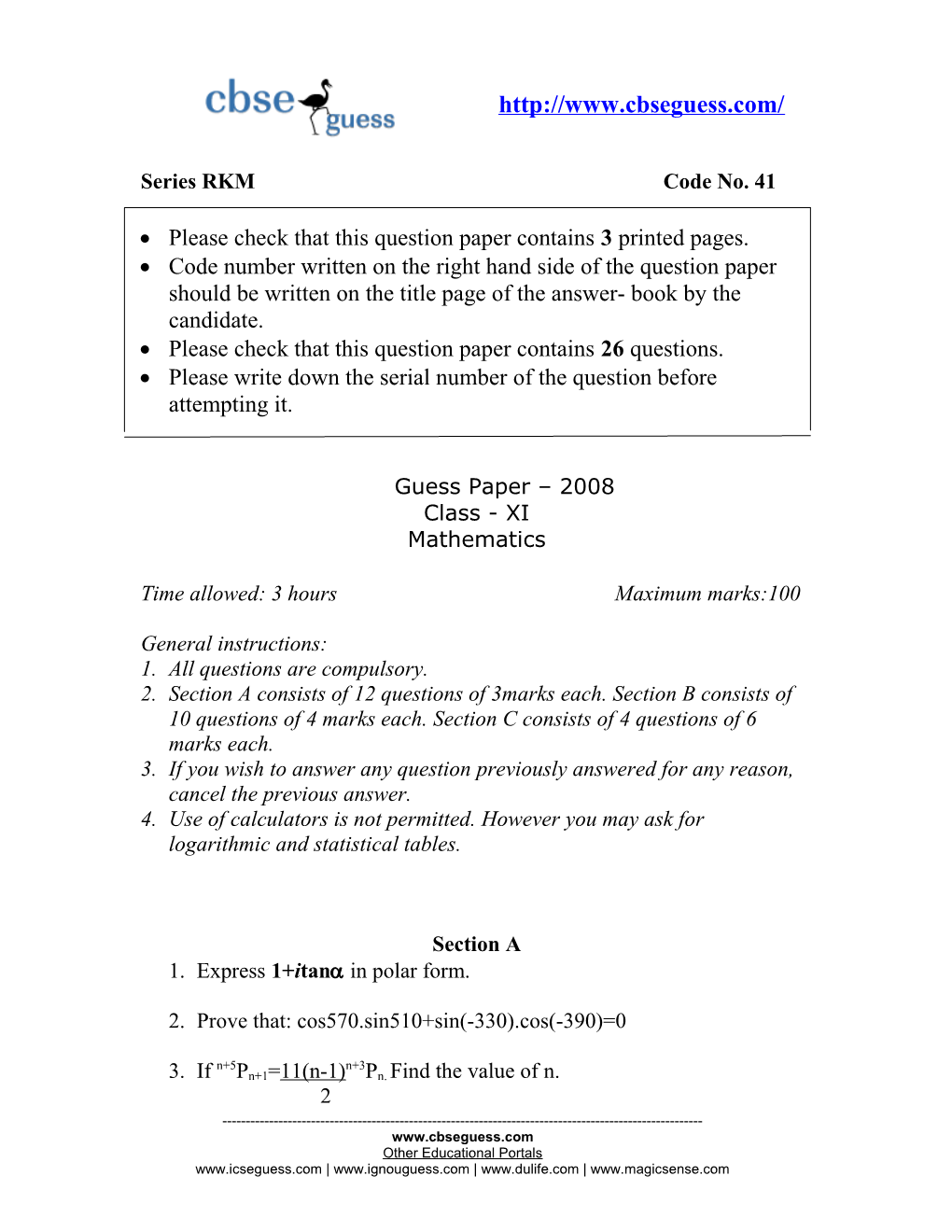# Series RKM Code No. 41/

Series RKM Code No. 41

• Please check that this question paper contains 3 printed pages.
• Code number written on the right hand side of the question paper should be written on the title page of the answer- book by the candidate.
• Please check that this question paper contains 26 questions.
• Please write down the serial number of the question before attempting it.

Guess Paper – 2008

Class - XI

Mathematics

## Time allowed: 3 hours Maximum marks:100

General instructions:

1. All questions are compulsory.
2. Section A consists of 12 questions of 3marks each. Section B consists of 10 questions of 4 marks each. Section C consists of 4 questions of 6 marks each.
3. If you wish to answer any question previously answered for any reason, cancel the previous answer.
4. Use of calculators is not permitted. However you may ask for logarithmic and statistical tables.

### Section A

1. Express 1+itan in polar form.
1. Prove that: cos570.sin510+sin(-330).cos(-390)=0
1. If n+5Pn+1=11(n-1)n+3Pn. Find the value of n.

2

1. Prove that: sin100sin500sin600sin700= √3

16

1. The longest side of triangle is three times the shortest side and the third side is 2cm shorter than the longest side. If perimeter of the triangle is at least 61cm, find the minimum length of the shortest side.
1. Solve for x: x2-(32-2i)x-62i=0

5

1. Evaluate: 20C5+25-rC4

r=2

1. Find the angle between the minute hand of a clock and the hour hand when the time is 7:20AM.
1. Find the sum of 5+55+555+5555+… upto n terms.
1. The sum of two numbers is 13/6. An even number of arithmetic means are being inserted between them and their sum exceeds their number by1. Find the number of means inserted.
1. If tan(cos)=cot(sin), prove that cos - = 1

4 22

1. If sin( +) = 1-m, prove that: tan - tan - =m

cos(-) 1+m 4 4

### Section B

1. Solve graphically the following system of inequations:

3x+2y<24, x+2y<16, x+y<10, x>0, y>0

1. How many different words can be formed with the letters of the word HARYANA? How many of these begin with H and end with N?In how many of these H and N are together?
1. There are 10 points in a plane, no three of which are in the same straight line except 4 points, which are collinear. Find the (i) number of straight lines obtained from the pairs of these points, (ii) number of triangles that can be formed with the vertices as these points.
1. Prove by the principal of mathematical induction that

2.7n+3.5n-5 is divisible by 24 for all n belongs to N.

1. Find the sum to n terms of the series:

12+(12+22)+(12+22+32)+.....

1. The number of terms of an AP is even, the sum of the odd terms is 24, of the even terms is 30, and last term exceeds the first term by 10 ½, find the number of terms and the series.
1. How many natural numbers not exceeding 4321 can be formed with the digits 1,2,3 and 4, if the digits can repeat?
1. Prove by the principle of mathematical induction that

7+77+777+....+777...... 7=7(10n+1-9n-10)

n- digits 81

1. Solve for x: √3cosθ+sinθ=√2
1. Prove that:

CosA cos2A cos22A cos23A...... cos2n-1A=sin2nA

2nsinA

Section C

1. If S1, S2 and S3 be respectively the sum of n, 2n and 3n terms of a GP, prove that S1(S3-S2)=(S2-S1)2 .
1. Prove that: sin5A=5cos4AsinA-10cos2Asin3A+sin5A
1. Express the following in polar form:

(Cosx+isinx)(cosy+isiny)

(cot u +i)(1+ i tan u)

1. For any triangle ABC, prove that:

cosA+cosB+cosC=4 cosB+C cosC+A cosA+B

2 2 2 2 2 2

------

Other Educational Portals
| | |